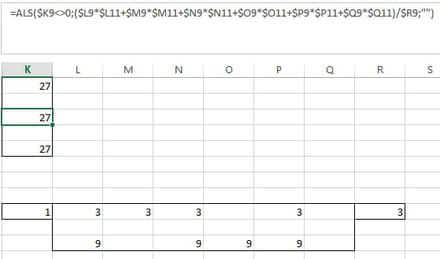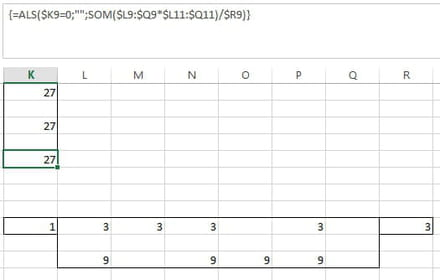How to simply this Excel formula

Solved/Closed
-
Posts
2847
Registration date
Sunday September 12, 2010
Status
Moderator
Last seen
January 13, 2022
-
Is there a way to make this formula simpler?

=IF(\$K9=0,"",SUM(\$L9*\$L11)/R9+IF(\$K9=0,"",SUM(\$M9*\$M11)/\$R9+IF(\$K9=0,"",SUM(\$N9*\$N11)/\$R9+IF(\$K9=0,"",SUM(\$O9*\$O11)/\$R9+IF(\$K9=0,"",SUM(\$P9*\$P11)/\$R9+IF(\$K9=0,"",SUM(\$Q9*\$Q11)/\$R9))))))

Posts
2847
Registration date
Sunday September 12, 2010
Status
Moderator
Last seen
January 13, 2022
491
Hi Rufus,

=IF(\$K9<>0,(\$L9*\$L11+\$M9*\$M11+\$N9*\$N11+\$O9*\$O11+\$P9*\$P11+\$Q9*\$Q11)/\$R9,"")

Or even shorter:
=IF(\$K9=0,"",SUM(\$L9:\$Q9*\$L11:\$Q11)/\$R9)
NOTE: This is an array formula and needs to be confirmed using Ctrl+Shift+Enter. When done correctly the formula will be enclosed by curly brackets { }.

Best regards,
Trowa

Thanks Trowa, That worked great. However if for instance Q9 and Q11 is blank it returns a #VALUE!. I need the formula to ignore the blanks. I am using Microsoft Office 2019.
0
Posts
2847
Registration date
Sunday September 12, 2010
Status
Moderator
Last seen
January 13, 2022
491
Hi Rufus,

Not sure why that is, as it doesn't happen to me:

Formula1:Formula2:NOTE: I'm using a Dutch version:
ALS translates as IF
SOM as SUM
; as ,

Do you maybe notice a difference between my test setup and your data?

Best regards,
Trowa
0